## Differentation Rules

Many differentiation rules can be proven using the limit definition of the derivative and are also useful in finding the derivatives of applicable functions. To eliminate the need of using the formal definition for every application of the derivative, some of the more useful formulas are listed here.

1. If f( x) = c, where c is a constant, the f′ ( x) = 0.

2. If f( x) = c · g( x), then f′ ( x) = c · g′ ( x).

3. Sum Rule: If f( x) = g( x) + h( x), then f′ ( x) = g′ ( x) + h′ ( x).

4. Difference Rule: If f( x) = g( x) − h( x), then f′ ( x) = g′ ( x) − h′ ( x).

5. Product Rule: If f( x) = g( x) · h( x), then f′ ( x)= g′ ( x) · h( x)+ h′ ( x) · g( x).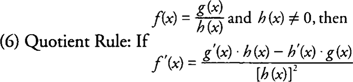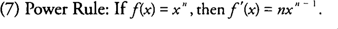Example 1: Find f′ ( x) if f ( x) = 6 x 3 + 5 x 2 + 9.Example 2: Find y′ if y = (3 x + 4)(2 x 2 − 3 x + 5).Example 3: Find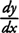if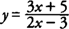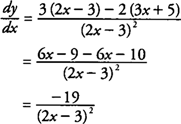Example 4: Find f′( x) ifExample 5: Find f′(3) if f ( x) = x 2 − 8 x +3.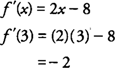Example 6: If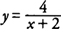, find y′ at (2,1).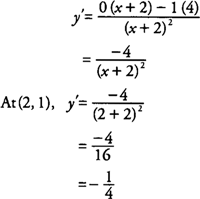Example 7: Find the slope of the tangent line to the curve y = 12 − 3 x 2 at the point (−1,9).

Because the slope of the tangent line to a curve is the derivative, you find that y′ = −6 x; hence, at (−1,9), y′ = 6, and the tangent line has slope 6 at the point (−1,9).